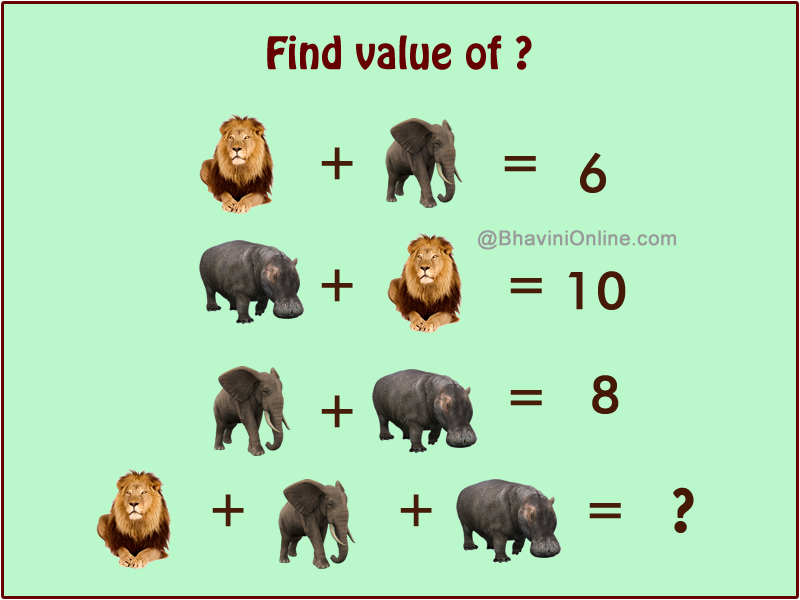# Fun Math Riddle: Lion + Elephant + Hippo = ?

Here’s some easy level basic Maths Riddle.

Look at the pictures and find the sum of the animals shown in the image.

To be represent the equation in words, we can write;

Lion + Elephant = 6

Hippo + Lion = 10

Elephant + Hippo = 8

Lion + Elephant + Hippo = ?You should remember this kind of equations from your childhood.

To make learning fun numbers are often replace by animals or things so that it has a visual appeal.

Check your friends maths skills by sending them this riddle on WhatsApp and Facebook.

Do they remember basic Maths? You will find out.

We have 3 equations;

Lion + Elephant = 6

Hippo + Lion = 10

Elephant + Hippo = 8

Adding all 3 of them we get;

2(lion) + 2(elephant) + 2(hippo) = 24

Solving it we get;

2(lion + elephant + hippo) = 24

(lion + elephant + hippo) = 24/2 = 12

Therefore;

Lion + Elephant + Hippo = 12